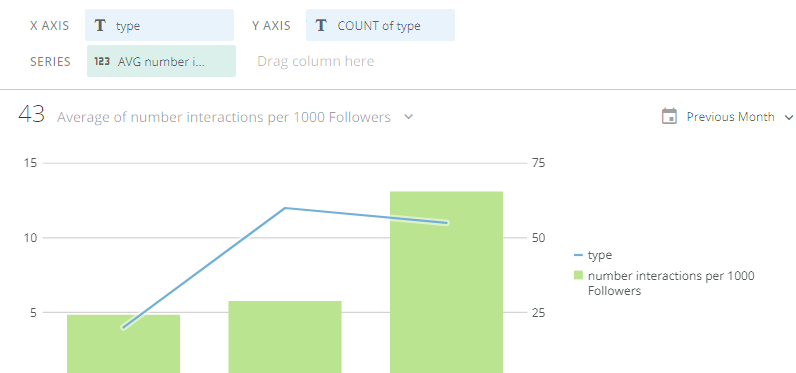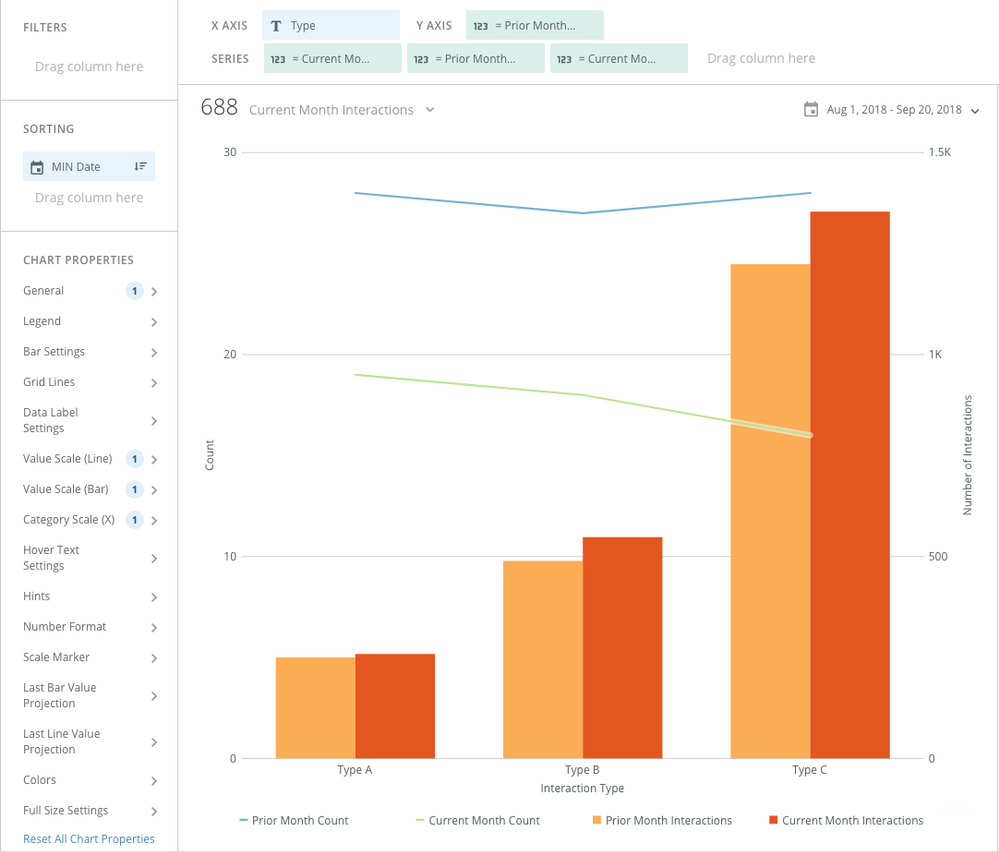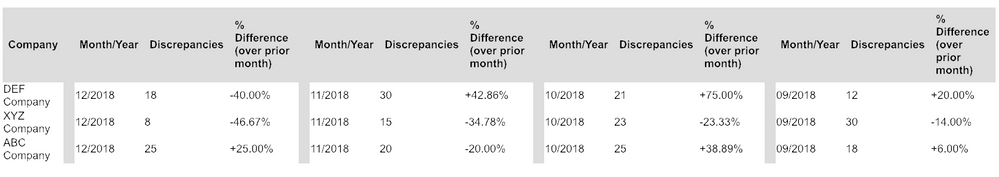# hello , how to compare month to month with secondary axis in card visualization?

hello , how to compare month to month with secondary axis in card visualization? for example i want to compare 2 months• Hi, user04753,

If I understand your question correctly, you want a card that has a month-over-month comparison of two sets of metrics (count; avg interactions) that will appear on two different y axis scales, broken out by Type on the x axis. Is that correct?

I think you can do this using a Beast Mode. Check out this Knowledgebase article that explains some general concepts and give examples: http://knowledge.domo.com?cid=beastmodecomparison

Since you effectively want to include current and prior month count and average number of interactions (a total of 4 metrics), you'll need to create each of those metrics as a separate beast mode. That's because of the way the "Line + Grouped Bar" chart works.

The logic in these beast modes is to count or average the interactions if the month of the date is the same as the month of the current date (current month) or if the month of date + 1 month is the same as the month of the current date (prior month).

The four beast modes:

1) Current Month Count:

`count(  case     when month(`Date`) = month(CURRENT_DATE()) then `Type`  end)`

2) Prior Month Count:

`count(  case     when month(date_add(`Date`,interval 1 month)) = month(CURRENT_DATE()) then `Type`  end)`

3) Current Month Interactions:

`avg(  case     when month(`Date`) = month(CURRENT_DATE()) then `Number of Interactions`  end)`

4) Prior Month Interactions:

`avg(  case     when month(date_add(`Date`,interval 1 month)) = month(CURRENT_DATE()) then `Number of Interactions`  end)`

Create the visualization:

To create the visualization, you'll put "Type" on the X axis, then add "Prior Month Count" as the Y axis, followed by "Current Month Count", "Prior Month Interactions", and "Current Month Interactions" as series.

By default, this chart will plot the field in the Y axis as a line and will plot each of the three series as bars. You can change that defualt behavior by changing this parameter: Chart Properties --> General --> Series on Left Scale. By entering the value 2, you're telling Domo to plot the first two fields as lines and all remaining fields as bars.

Your chart will look something like this:• I think that you would need to make these changes in SQL or an ETL.

Basically, you would need to add a field to your data set for `Previous Month Date` this would just be

SELECT

*,

DATESUB(`Date_Field`, INTERVAL 1 MONTH) as `Previous Month Date`

FROM data_table

You would the new table with the original table

SELECT

a.*,

b.`type` as `Previous Month Type`,

b.`number interactions` as `Previous Month number interactions`

from new_table a

left join data_table b

on a.`unique ID`=b.`Unique ID` AND a.`Previous Month Date`=b.`Date`

This should give you a table that includes the current months value and the previous months value in the same line.

Then you would add the two new fields to your visualization: type, previous month type, number interactions, previous month number interactions.

Set the left axis to include 2 fields and you should be all set.  Let me know how this goes.  Good luck

• UPDATE: did start a new thead as well since this may not be seen by others who might've been looking to find a solution to something similar. Thanks!

https://dojo.domo.com/t5/Beast-Mode-ETL-Dataflow/Table-compare-several-month-over-month/m-p/38216#M6274

I was going to start a new thread but this sounds like it might be along the lines of what we're looking to do but in a table, so wondering if either of these solutions (beast mode or SQL/ETL) can accommodate MORE than 2 dates in a single row of data to compare several months, showing total discrepanices along with the percentage difference from the previous month. The month over month bar chart doesn't seem to be able to visualize what we need, and hoped maybe it can in a table.

EXAMPLE:We want to show, from left to right,  CURRENT MONTH (December) data w/PRIOR MONTH (November) percentage variance, then November compared to October, & October compared to September. The column to the left would be by company/organization along w/current month, # of discrepanices, & the % change over the previous month. Then it would continue, left to right, for the previous 3 months from the current month.

Can this be done?